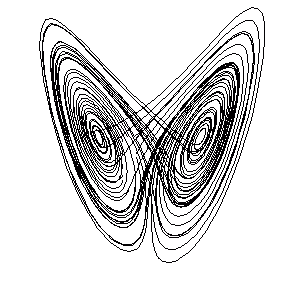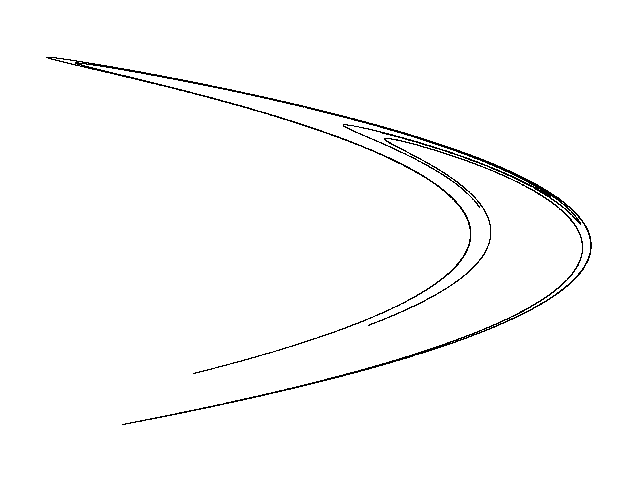# Research Interests

My main interest is the study of ergodic theory and its applications. Below an overview of the basic ideas in ergodic theory is given, followed by some specific research interests. See also my papers/preprints here for formal details of results.

• Dynamical Systems and Ergodic Theory.
• Statistical Properties of Lorenz Attractors.
• Ergodic Theory of Strange Attractors.

## Dynamical Systems and Ergodic Theory

Basic ideas. Given a discrete time map f:X-> X, of a space X into itself, and preserving a measure m (so that m(f-1}A)=m(A) for all subsets A &sub X) ergodic theory provides a mechanism of understanding the limiting behaviour of the time averages:

1/n{Q(x)+Q(fx)+...Q(fn-1x)},

for some given x in X and as time n goes to infinity . Here Q:X->R denotes a real-valued observation on the system (for example an indicator function). We refer to a (f,X,m) ergodic system as one which is dynamically indecomposable: all f-invariant sets in X have zero m-measure. This implies that m-almost all orbits in X are dense. For purposes of applications, an important result is the Birkhoff Ergodic Theorem which states that space and time averages are equal. That is:
Limitn{1/n(Q(x)+Q(fx)+...Q(fn-1x))}= &int Q(x) dm(x), (modulo zero m-measure x&isin X)

Simple examples of ergodic systems. The coin tossing map (or Bernoulli map) f:[0,1]->[0,1] given by f(x)=2x (mod 1) is ergodic and the invariant measure is Lebesgue measure. Another example is an irrational rotation of the circle, i.e the map r:[0,1]->[0,1] given by r(x)=x+&alpha (mod 1), with &alpha an irrational number.

Mixing ergodic systems. A mixing dynamical system is one for which future observations performed on the system are (asymptotically) independent of those performed in the past. Formally we say (f,X,m) is a mixing system if

Limitn m(f-nA &cap B) = m(A)m(B)
for all m-measurable A nd B. The coin tossing map is mixing but the irrational rotation map is not mixing. From the definitions notice that mixing actually implies ergodicity. The rate of mixing is also of interest and one would like to measure the (asymptotic) decay of the quantity
&int P(fn(x))Q(x) dm - &int P(x) dm &int Q(x) dm
as n goes to infinity. This quantity is known as a "correlation" or "covariance" function where P and Q are some general functions. If P and Q are sufficiently smooth functions (for example Lipshitz continuous) then one can get good asymptotics for the rate of decay of correlations. For the Bernoulli map the rate of decay is exponentially fast.

### Statistical properties of Lorenz attractors.

The classical Lorenz for parameters &sigma=10, &beta=8/3 and &rho=28 are given by:
dx/dt = &sigma (y-x)
dy/dt = &rho x - y - xz
dz/dt = xy - &beta z.
In 1963, these equations where studied by E. Lorenz as a model for fuild convenction, and it was observed that these equations gave rise to chaotic behaviour: nearby initial conditions quickly diverge, and future flow trajectories do not settle down to some simple attracting set. In fact all flow trajectories traced out a complicated set which resembled a butterfly: a "strange attractor" was observed. See below.The Lorenz strange attractor

Rigorous studies have shown that the Lorenz attractor is indeed "strange" rather than a simple periodic orbit, and furthermore the attractor is not destroyed if the parameters of the system are slightly changed. The dynamics on the attractor can also be described probabilistically: the asymptotic evolution of typical initial trajectories are governed by an ergodic measure (called a Sinai-Ruelle-Bowen measure). Thus in effect every initial condition distributes itself over the attractor in a "roughly uniform" way. "Roughly uniform" in the sense that the invariant probability density on the attractor is comparable to a uniform density. Moreover it is known that Lorenz equations are mixing with respect to this measure.
Related problems for which I am active in working on include understanding the rate of mixing for the Lorenz flow itself, and whether the Lorenz flow satisfies any statistical limit laws (such as the Central Limit Theorem). One can ask the same questions for other parameters, such as those close to &rho=32. In this parameter regime the attractor is not robust: slight changes in parameter destroy the strangeness. However it is hoped that "strangeness" together with the associated ergodic properties are preserved for a positive measure subset of these parameters.

### Ergodic theory of strange attractors.

The Hénon map f:(x,y)->(a-x2+y, bx) with classical parameters a=1.3 and b=0.4 was studied first in 1974 by astronomer M. Hénon. For these parameters it is still an open problem to decide whether the observed attractor is genuinely strange or whether it is just some high period attractor.The Hénon strange attractor

To support the latter, work by Newhouse in the 70's suggests that the existence of high periodic attractors is a generic phenomenon in the paramter space of such examples. On a technical level this is a consequence of having persistent homoclinic tangencies between stable and unstable manifolds. Choatic sets in the sense of the Smale Horsehoe map also coexist but they occupy zero area of the phase space, and thus would not be observed numerically. On the other hand work in the 90's by Benedicks-Carleson demonstrate that for a positive probability choice of parameters genuine strange attractors exist (for b small and a close to 2). Ergodic properties of Hénon maps for the Benedicks-Carleson parameters are now also known: Sinai-Ruelle-Bowen measures exist and Hénon maps mix exponentially fast with respect to these ergodic measures.
Related open problems would be to understand the ergodic properties of non-smooth Hénon-like maps. Such maps arise naturally as Poincaré maps in nonlinear ODE's (for example the Lorenz equations).